Weve seen how to make lists and arrays with Table. Theres also a way to do it using pure functionswith Array.
Generate a 10-element array with an abstract function f:
 In:=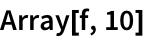Out=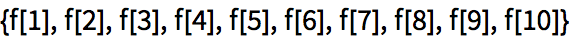In:=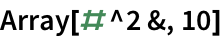Out=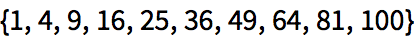Table can give the same result, though you have to introduce the variable n:
 In:=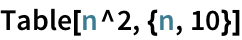Out=Array[f,4] makes a single list of 4 elements. Array[f,{3,4}] makes a 3×4 array.
Make a list of length 3, each of whose elements is a list of length 4:
 In:=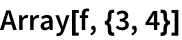Out=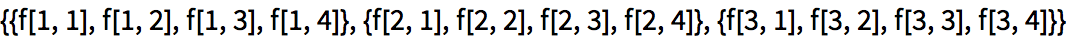In:=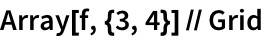Out=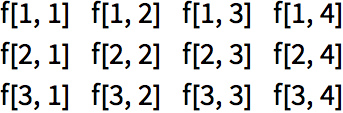If the function is Times, Array makes a multiplication table:
 In:=Out=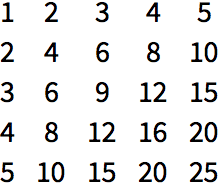What if we want to use a pure function in place of Times? When we compute Times[3,4], we say that Times is applied to two arguments. (In Times[3,4,5], Times is applied to three arguments, etc.) In a pure function, #1 represents the first argument, #2 the second argument and so on.
#1 represents the first argument, #2 the second argument:
 In:=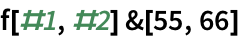Out=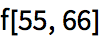#1 always picks out the first argument, and #2 the second argument:
 In:=Out=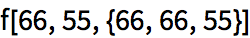Now we can use #1 and #2 inside a function in Array.
Use a pure function to make a multiplication table:
 In:=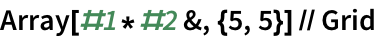Out=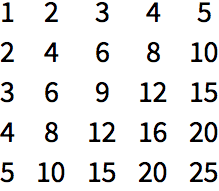Use a different pure function that puts in x whenever the numbers are equal:
 In:=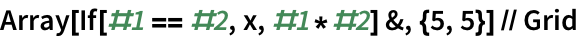Out=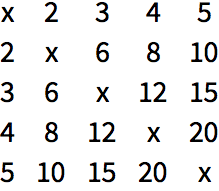Heres the equivalent computation with Table:
 In:=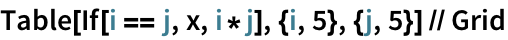Out=NestList takes a single function, say f, and successively nests it:
 In:=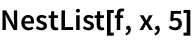Out=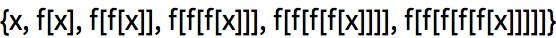In:=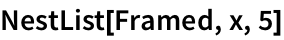Out=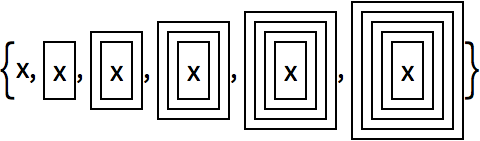NestList just keeps on applying a function to whatever result it got before. FoldList does the same, except that it also folds in a new element each time.
Heres FoldList with an abstract function f:
 In:=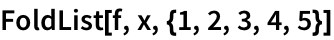Out=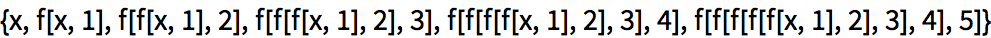Including Framed makes it a little easier to see whats going on:
 In:=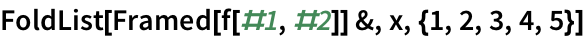Out=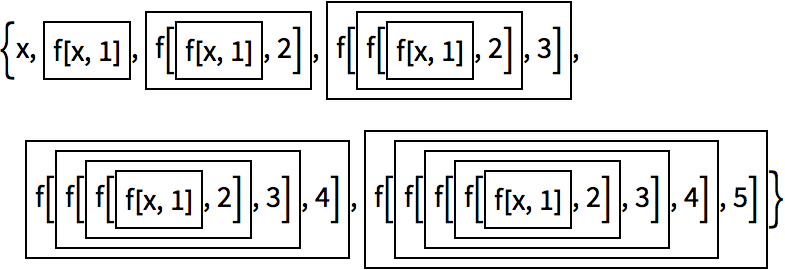At first, this may look complicated and obscure, and it might seem hard to imagine how it could be useful. But actually, its very useful, and surprisingly common in real programs.
FoldList is good for progressively accumulating things. Lets start with a simple case: progressively adding up numbers.
At each step FoldList folds in another element (#2), adding it to the result so far (#1):
 In:=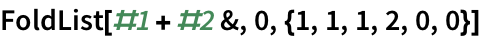Out=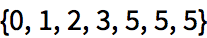Heres the computation its doing:
 In:=Out=Or equivalently:
 In:=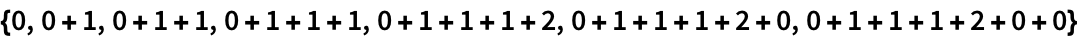Out=In:=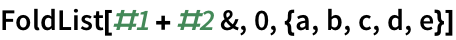Out=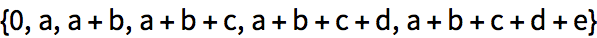Of course, this case is simple enough that you dont actually need a pure function:
 In:=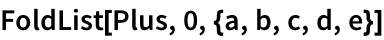Out=A classic use of FoldList is to successively fold in digits to reconstruct a number from its list of digits.
Successively construct a number from its digits, starting the folding process with its first digit:
 In:=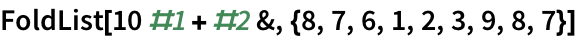Out=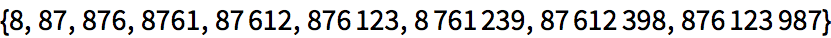Finally, lets use FoldList to fold in progressive images from a list, at each point adding them with ImageAdd to the image obtained so far.
Progressively fold in images from a list, combining them with ImageAdd:
 In:=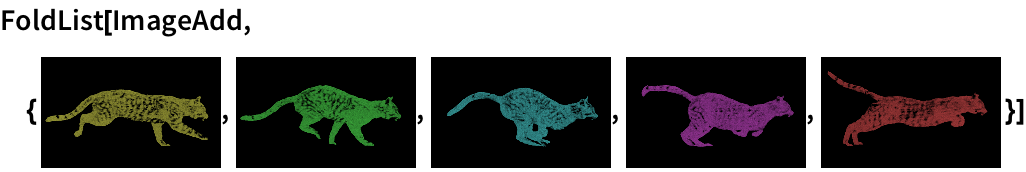Out=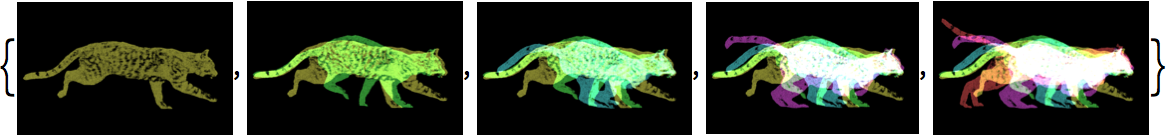The concept of FoldList is not at first the easiest to understand. But when youve got it, youve learned an extremely powerful functional programming technique thats an example of the kind of elegant abstraction possible in the Wolfram Language.
29.1Use Prime and Array to generate a list of the first 100 primes. »
Expected output:
 Out[]=Answer & check your solution
29.2Use Prime and Array to find successive differences between the first 100 primes. »
Expected output:
 Out[]=Answer & check your solution
29.3Use Array and Grid to make a 10 by 10 addition table. »
Expected output:
 Out[]=Answer & check your solution
29.4Use FoldList, Times and Range to successively multiply numbers up to 10 (making factorials). »
Expected output:
 Out[]=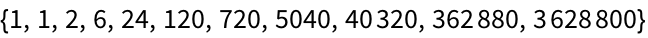Answer & check your solution
29.5Use FoldList and Array to compute the successive products of the first 10 primes. »
Expected output:
 Out[]=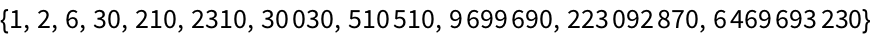Answer & check your solution
29.6Use FoldList to successively ImageAdd regular polygons with between 3 and 8 sides, and with opacity 0.2. »
Expected output:
 Out[]=Answer & check your solution
+29.1Use Array to make a 10 by 10 grid where the leading diagonal and everything below it is 1 and the rest is 0. »
Expected output:
 Out[]=Answer & check your solution
What does # alone mean?
Its equivalent to #1a slot to be filled from the first argument of a function.
How does one say #1?
Can one name arguments to a pure function?
Yes. Its done with Function[{x, y}, x+y], etc. Its sometimes nice to do this for code readability, and its sometimes necessary when pure functions are nested.
Can Array make more deeply nested structures?
Yesas deep as you want. Lists of lists of lists... for any number of levels.